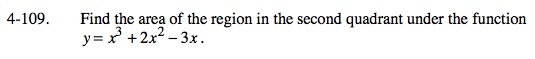### Home > CALC > Chapter 4 > Lesson 4.3.2 > Problem4-109

4-109.To set up the integral, first determine the bounds in which this function exists in the 2nd quadrant.

Determine the roots:
0 = x3 + 2x2 − 3x
0 = x(x2 + 2x − 3) = x(x + 3)(x − 1)
Roots: x = 0, x = −3, and x = 1
$\int_{-3}^0(x^3+2x^2-3x)dx=$